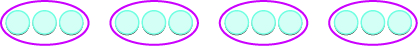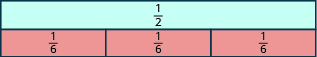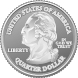## Dividing Fractions

### Learning Outcomes

• Use a model to describe the result of dividing a fraction by a fraction
• Use an algorithm to divide fractions

Why is $12\div 3=4?$ We previously modeled this with counters. How many groups of $3$ counters can be made from a group of $12$ counters?There are $4$ groups of $3$ counters. In other words, there are four $3\text{s}$ in $12$. So, $12\div 3=4$.
What about dividing fractions? Suppose we want to find the quotient: $\Large\frac{1}{2}\normalsize\div\Large\frac{1}{6}$. We need to figure out how many $\Large\frac{1}{6}\normalsize\text{s}$ there are in $\Large\frac{1}{2}$. We can use fraction tiles to model this division. We start by lining up the half and sixth fraction tiles as shown below. Notice, there are three $\Large\frac{1}{6}$ tiles in $\Large\frac{1}{2}$, so $\Large\frac{1}{2}\normalsize\div\Large\frac{1}{6}\normalsize=3$.### Example

Model: $\Large\frac{1}{4}\normalsize\div\Large\frac{1}{8}$

Solution:
We want to determine how many $\Large\frac{1}{8}\normalsize\text{s}$ are in $\Large\frac{1}{4}$. Start with one $\Large\frac{1}{4}$ tile. Line up $\Large\frac{1}{8}$ tiles underneath the $\Large\frac{1}{4}$ tile.There are two $\Large\frac{1}{8}$s in $\Large\frac{1}{4}$.
So, $\Large\frac{1}{4}\normalsize\div\Large\frac{1}{8}\normalsize=2$.

### Try It

Model: $\Large\frac{1}{3}\normalsize\div\Large\frac{1}{6}$

Model: $\Large\frac{1}{2}\normalsize\div\Large\frac{1}{4}$

The following video shows another way to model division of two fractions.

### Example

Model: $2\div\Large\frac{1}{4}$

### Try It

Model: $2\div\Large\frac{1}{3}$

Model: $3\div\Large\frac{1}{2}$

The next video shows more examples of how to divide a whole number by a fraction.

Let’s use money to model $2\div\Large\frac{1}{4}$ in another way. We often read $\Large\frac{1}{4}$ as a ‘quarter’, and we know that a quarter is one-fourth of a dollar as shown in the image below. So we can think of $2\div\Large\frac{1}{4}$ as, “How many quarters are there in two dollars?” One dollar is $4$ quarters, so $2$ dollars would be $8$ quarters. So again, $2\div\Large\frac{1}{4}\normalsize=8$.

The U.S. coin called a quarter is worth one-fourth of a dollar.Using fraction tiles, we showed that $\Large\frac{1}{2}\normalsize\div\Large\frac{1}{6}\normalsize=3$. Notice that $\Large\frac{1}{2}\cdot \frac{6}{1}\normalsize=3$ also. How are $\Large\frac{1}{6}$ and $\Large\frac{6}{1}$ related? They are reciprocals. This leads us to the procedure for fraction division.

### Fraction Division

If $a,b,c,\text{ and }d$ are numbers where $b\ne 0,c\ne 0,\text{ and }d\ne 0$, then

$\Large\frac{a}{b}\normalsize\div\Large\frac{c}{d}=\frac{a}{b}\cdot \frac{d}{c}$

To divide fractions, multiply the first fraction by the reciprocal of the second.

We need to say $b\ne 0,c\ne 0\text{ and }d\ne 0$ to be sure we don’t divide by zero.

Tip:  Here’s a rhyme to help you with dividing fractions.  When dividing fractions don’t ask why, just flip the second and multiply.

### Example

Divide, and write the answer in simplified form: $\Large\frac{2}{5}\normalsize\div\Large\left(-\frac{3}{7}\right)$

### Try It

Watch this video for more examples of dividing fractions using a reciprocal.

### Example

Divide, and write the answer in simplified form: $\Large\frac{2}{3}\normalsize\div\Large\frac{n}{5}$

### Example

Divide, and write the answer in simplified form: $\Large-\frac{3}{4}\normalsize\div\Large\left(-\frac{7}{8}\right)$

### Try It

The following video shows more examples of dividing fractions that are negative.

### Example

Divide, and write the answer in simplified form: $\Large\frac{7}{18}\normalsize\div\Large\frac{14}{27}$